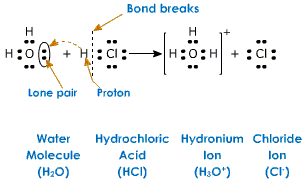# Biochemistry Review Questions - pH, Buffer, Ionization of water, Weak acids, and Weak bases

16. A hydronium ion:a) has the structure H3O+.
b) is a hydrated hydrogen ion.
c) is a hydrated proton.
d) is the usual form of one of the dissociation products of water in solution.
e) all of the above are true.

17. The pH of a solution of 1 M HCl is:
a) 0
b) 0.1
c) 1
d) 0.01
e) –1

18. Which of the following is true about the properties of aqueous solutions?
a) A pH change from 5.0 to 6.0 reflects an increase in the hydroxide ion concentration ([OH-]) of 20%.
b) A pH change from 8.0 to 6.0 reflects a decrease in the proton concentration ([H+]) by a factor of 100.
c) Charged molecules are generally insoluble in water.
d) Hydrogen bonds form readily in aqueous solutions.
e) The pH can be calculated by adding 7 to the value of the pOH.

19. The pH of a sample of blood is 7.4, while gastric juice is pH 1.4. The blood sample has:
a) 0.189 times the [H+] as the gastric juice.
b) 5.29 times lower [H+] than the gastric juice.
c) 6 times lower [H+] than the gastric juice.
d) 6,000 times lower [H+] than the gastric juice.
e) a million times lower [H+] than the gastric juice.

20. The aqueous solution with the lowest pH is:
a) 0.01 M HCl.
b) 0.1 M acetic acid (pKa = 4.86).
c) 0.1 M formic acid (pKa = 3.75).
d) 0.1 M HCl.
e) 10–12 M NaOH.

21. Phosphoric acid is tribasic, with pKa’s of 2.14, 6.86, and 12.4. The ionic form that predominates at pH 3.2 is:
a) H3PO4.
b) H2PO4.
c) HPO42–.
d) PO43–.
e) none of the above.

22. In which reaction below does water not participate as a reactant (rather than as a product)?
a) Conversion of an acid anhydride to two acids.
b) Conversion of an ester to an acid and an alcohol.
c) Conversion of ATP to ADP.
d) Photosynthesis
e) Production of gaseous carbon dioxide from bicarbonate.

23. Which of the following properties of water does not contribute to the fitness of the aqueous environment for living organisms?
a) Cohesion of liquid water due to hydrogen bonding.
b) High heat of vaporization.
c) High specific heat.
d) The density of water is greater than the density of ice.
e) The very low molecular weight of water.

24.Which of the following statements about buffers is true?
a) A buffer composed of a weak acid of pKa = 5 is stronger at pH 4 than at pH 6.
b) At pH values lower than the pKa, the salt concentration is higher than that of the acid.
c) The pH of a buffered solution remains constant no matter how much acid or base is added to the solution.
d) The strongest buffers are those composed of strong acids and strong bases.
e) When pH = pKa, the weak acid and salt concentrations in a buffer are equal.

25 A compound has a pKa of 7.4. To 100 mL of a 1.0 M solution of this compound at pH 8.0 is added 30 mL of 1.0 M hydrochloric acid. The resulting solution is pH:
a) 6.5
b) 6.8
c) 7.2
d) 7.4
e) 7.5
16. e) all of the above are true.
17. a) 0
18. d) Hydrogen bonds form readily in aqueous solutions.
19. e) a million times lower [H+] than the gastric juice.
20. d) 0.1 M HCl.
21. b) H2PO4.
22. e) Production of gaseous carbon dioxide from bicarbonate.
23. e) The very low molecular weight of water.
24. e) When pH = pKa, the weak acid and salt concentrations in a buffer are equal.
25. d) 7.4

Reference: Lehninger Principles of Biochemistry Lecture Notebook 4th Revised Edition
(Chapter 2 Water)

1.2.